## Monday, January 27, 2014

### Predictive Analytics in Tableau Part 5: Polynomial, Exponential and Piecewise Regression

Today, we will talk about some more types of regression using Tableau 8.1's new R functionality.  We previously talked about prediction using Linear Regression.  But, what if the relationship isn't linear?  In the real world, linearity is rarely the truth.  For this demonstration, we will use the same data set we used in Parts 1, 2, and 3.

First, let's look at our data.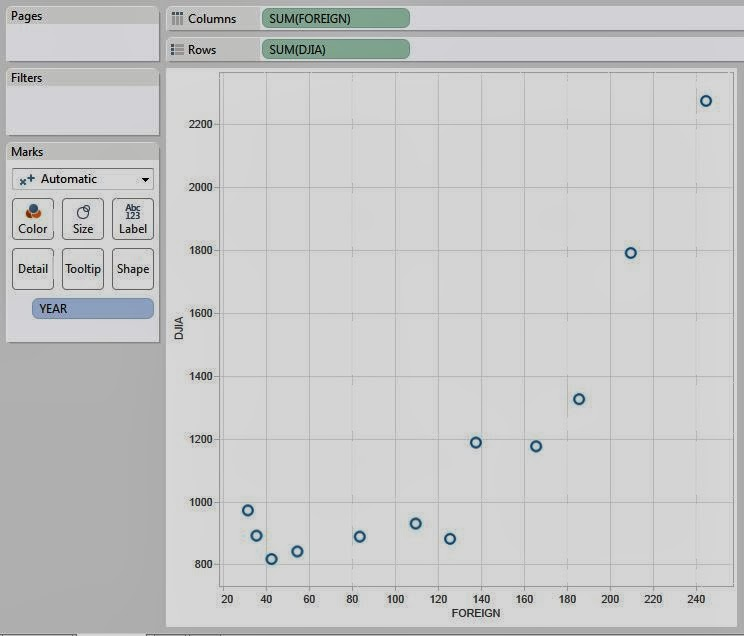DJIA vs. Foreign by Year
As you can see, this data is not related linearly.  There is very little change in DJIA when Foreign is below 140.  However, when Foreign surpasses 140, DJIA skyrockets.  If we tried to use a line to predict these values, we would get something like this:DJIA vs. Foreign by Year (with Linear Trend)
This line is pretty far off from most of those points.  Fortunately, Tableau offers some more built-in trend lines.  Here are some good ones: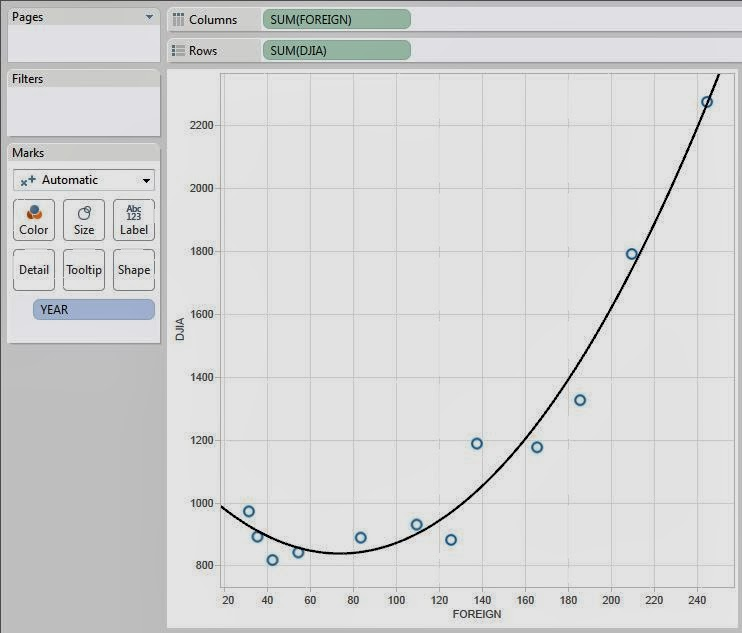DJIA vs. Foreign by Year (with Quadratic Trend)DJIA vs. Foreign by Year (with Exponential Trend)
As you can see, both of these models fit the data pretty well.  Now, let's see how to do them using R.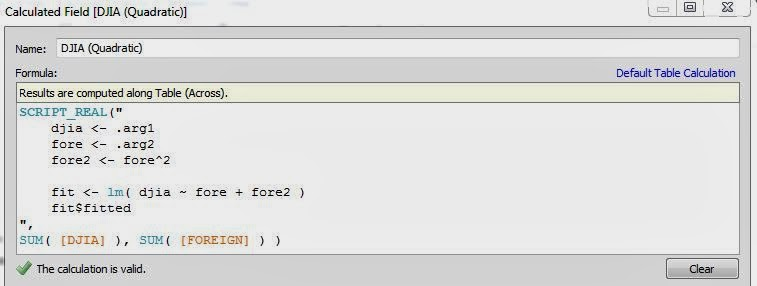DJIA (Quadratic)DJIA (Exponential)
We see that all we have to do is make a new variable with whatever function we want and add it to the model.  As far as R knows, these are two completely different variables.  We could add all of the other variables to the mix as well if we wanted to.  We could easily model Foreign as a quadratic while modeling Consumer as a logarithm.  One important thing to note is that if you include a polynomial, you should include all lesser degrees.  Simply put, if you have a x^2, then you must also have x.  If you have x^3, you must also have x^2 and x.  We won't go into detail about why you should do this at this time.

Looking back at the earlier trends, we're not happy with either of these trends.  The quadratic trend has that troubling curvature at the left side while the exponential trend doesn't seem strong enough to capture the upward curvature.  Now, let's make a new model that Tableau doesn't even have!

What if we believe that there are actually two trends here?  Let's imagine that the relationship is linear for small values of Foreign (less than 135) and a different linear relationship for larger values of Foreign?  No problem!  We can combine our models!

SCRIPT_REAL("
djia <- .arg1
fore <- .arg2
th <- 135
fore2 <- fore^2

smallfore <- fore[fore<th]
smalldjia <- djia[fore<th]

largefore <- fore[fore>=th]
largedjia <- djia[fore>=th]

smallfit <- lm( smalldjia ~ smallfore )
largefit <- lm( largedjia ~ largefore )

c(smallfit\$fitted, largefit\$fitted)
",
SUM( [DJIA] ), SUM( [FOREIGN] ) )

Finally, let's see the results.DJIA vs. Foreign by Year (with Piecewise Prediction)
This model fits our data so much better!  This method is called Piecewise Regression.  The amazing thing about R is that there's a method for predicting anything you want.  You can even create your own prediction method if you need to.  Thanks for reading.  We hope you found this informative.

P.S.

We're curious how Tableau keeps track of which value in the output vector corresponds to each input.  This was a huge obstacle in Part 3 as well.  If you have ideas, let us know in the comments.

Data Analytics Consultant
Mariner, LLC
llewellyn.wb@gmail.com

## Monday, January 20, 2014

### Predictive Analytics in Tableau Part 4: Logistic Regression

Today, we're going to talk about performing Logistic Regression using Tableau 8.1's R functionality.  Logistic Regression is very similar to Linear Regression, which we saw in the previous posts in this series.  However, Logistic Regression is designed to predict binary (Yes/No, 1/0) outcomes.  A very simple example is "Will this customer buy our product if we advertise to them?"  For this exercise, we will use the ubiquitous AdventureWorks data set from Microsoft.  If you've ever seen a Microsoft Data Mining demo, you've seen this data set.  Let's start by looking at our data.Customer Demographics
As you can see, we have quite a bit of information about our customers, as well as whether or not they purchased a bike from us in the past.  Now, let's look at a logistic model with only one predictor so that we can understand how it works.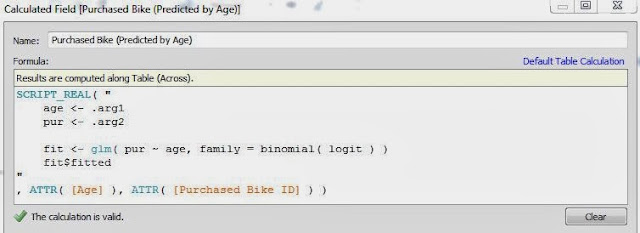Purchased Bike (Predicted by Age)
As you can see, this code is extremely similar to the code for creating a linear regression model.  The only differences are that this model uses a more complex function, glm(), and an extra parameter, family = binomial( logit ).  Now, let's see what the predictions look like.Predictions by Age
Some of you will immediately ask why this function returns decimals when we asked it to predict a Yes/No response.  Strictly speaking, logistic regression does not predict a Yes/No response, it predicts the probability of a particular response.  In other words, it tells us how likely this person is to buy a bike.  It's up to us to decide how we want to use these probabilities.  Let's look at these predictions in another way.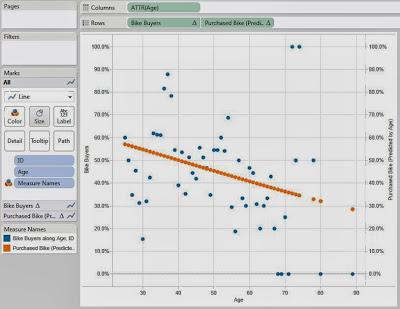Predictions by Age (Scatterplot)
As you can see, the probability of buying a bike decreases as the customer gets older.  This is an important, and not very surprising, discovery.  Now, how do we turn these probability into actual predictions?  That's up to us!  An easy way is to say "If the chance is greater than 50%, we say they will buy.  If it's less than 50%, we say they won't."  Let's see what this gets us.Predictions by Age (Classified)
This procedure doesn't seem to be very accurate.  Perhaps it's because we're only giving it one predictor.  Let's throw the rest of our variables in there and see if it gets better.Predictions (Classified)
These predictions are much better, but still not as accurate as we'd like.  Unfortunately, we couldn't find an easy way to look at these predictions in aggregate using this method.  So far, it seems that the new R implementation is really good for generating predictions.  However, it falls a little short when it comes to examining the model.  Fortunately, there's even more we could do here.  We could try a different model for this data, such as an artificial neural network or a bayesian model.  Maybe one of our readers can find a really neat way to display this data that sums it up nicely.  The rest is up to your imagination.  We hope that you found this informative.  Thanks for reading.

Data Analytics Consultant
Mariner, LLC
llewellyn.wb@gmail.com

## Monday, January 13, 2014

### Predictive Analytics in Tableau Part 3: Validating the Accuracy of your Predictions

Today, we will talk about validating the accuracy of your predictions using Tableau 8.1's R functionality.  In the previous posts in this series, we showed you how to create statistical models to predict values for your business.  However, we ignored a very important issue.  A model is supposed to be pretty good at predicting values that you used when you created it.  However, the true test of a model is how well it can predict values it doesn't know the answer to.  For this examination, we will use the same data set and design as we used in the first post in the series, Predictive Analytics in Tableau Part 1: Simple Linear Regression.

When you are creating a model, you typically want to "hold out" a portion of your data to use for testing later.  For instance, if we hold out 25% of our data, then we only use the remaining 75% when we created our model.  The 75% is known as the "Training Set" because it is used to train the model.  The 25% is known as the "Testing Set" because it is used to test the model after it has been trained.  First, let's see how you would create these sets.Consumer vs. Foreign
This is the data we have for creating our model.  As you can see, we only have 12 observations.  So, we would expect the training set to be 9 observations, and the testing set to be 3 observations.  Let's see how we would create this.Set
The code is not too complex.  Basically, it creates a 0/1 values for each year, with a 25% chance of 1 and 75% chance of 0.  Then, it changes the labels on these values to be more readable.  Now, let's see what it looks like on our chart.Consumer vs. Foreign (with Set)
As you can see, it put 8 observations in our training set, and 4 observations in the testing set.  This isn't exactly 25%, but it's only one observation off.  You should be aware that Tableau is going to query the R server every time you refresh this chart.  This means that the observations in the training set WILL change.  This is exactly the way we want it to work.  We want our training set to be as similar as possible to our testing set, with the exception of the size. Now, let's see how we could create our regression model using only our training set.  The code for "Foreign (Predicted)" is below:

SCRIPT_REAL( "
cons <- .arg1
fore <- .arg2
set <- .arg3

fore.train <- fore[set=='Training']
cons.train <- cons[set=='Training']

fit <- lm( fore.train ~ cons.train )
dat <- data.frame(cbind(fore, cons))
names(dat) <- c( 'fore.train', 'cons.train' )
predict(fit, dat, interval = 'prediction')[,1]
"
, SUM( [CONSUMER] ), SUM( [FOREIGN] ), [Set] )

This code simply uses the training data to create the model, then predicts values for all of the data.  Let's see this on our scatterplot.Consumer vs. Foreign (with Set, Prediction, and Trend)
This is just like the scatterplots we've been looking at, with a couple of additions.  First, we added our Regression model as the grey line.  Then, we added Tableau's Trend Line as the black line.  Our model was not trained using the testing data, but Tableau's trend line was.  So, what's the difference?  Not much according to this graph.  The lines are very close to one another.  This is one piece of evidence saying that this model predicts well.  Let's look at it differently.Consumer vs. Foreign (with Set, Prediction Interval, and Trend)
Here, we see that not only do all of the training and testing values fall within the bands, but so does the trend line.  This is a very good sign.  For those of you that are less graphical and more numeric, let's see this on a table.Consumer vs. Foreign (with Set, Prediction, and % Diff)
As you can see, our predictions can be off by as much as 35%.  You might ask "Why did we talk so highly about the model before when it predicts so poorly here?"  The answer is simple.  The model did the best it could with the amount of data it was given.  When you give a model ten values to look at, how accurate do you expect it to be?  The answer is not very.  However, in the business world, it's exceedingly rare to have extremely small data sets.  For instance, if you have monthly sales values for 5 years, that's 60 values.  You could easily hold out 10 or 15 of those and still get a good model.

There are no right answers when it comes to forecasting.  Everything is up to interpretation.  That's why so many companies put so much money into statisticians to develop accurate forecasts.  Even more to that point, perhaps linear regression isn't appropriate for this data set.  Maybe you would want to use a time series method, multiple regression, or a bayesian model.  Your predictive abilities are limited only by your data and your imagination.  We hope you found this informative.  Thanks for reading.

Associate Data Analytics Consultant
Mariner, LLC
llewellyn.wb@gmail.com

## Monday, January 6, 2014

### Predictive Analytics in Tableau Part 2: Linear Regression with Multiple Regressors

Today, we will talk about using Tableau 8.1's R functionality to perform predictive analysis via Multiple Regression.  In our previous post in the series, Predictive Analytics in Tableau Part 1: Simple Linear Regression, we talked about Simple Linear Regression.  Now, we're moving a step up and adding multiple variables to the mix.  However, we're going to keep it simple for now and keep all of the regressors at degree 1.  For those of you without advanced mathematics, which is probably most of you, a variable of degree 1 is linear, i.e. a straight line.  When the degrees get higher, the function get more curves.  If you want to know more, you can check out this article.  For this analysis, we will use the same data set as in the previous post, which can be found here.

To begin, let's look back at our scatterplot matrix again to see what our data looks like.
Initially, we can just throw everything against the wall and see what comes out.  We don't particularly care about knowing what the relationship is, we just want some predictions.  In these simple scenarios, it is safe to assume that more data is always better.  However, it's not always the case with real data.  But, we'll deal with that in a later post.  For now, let's just put everything in the model.  The code is too long to fit in one screenshot; so I have pasted it below:

SCRIPT_REAL( "

## Defining Variables

cons <- .arg1
crud <- .arg2
djia <- .arg3
fore <- .arg4
gnp <- .arg5
inte <- .arg6
purc <- .arg7

## Fitting the Model

fit <- lm( cons ~ crud + djia + fore + gnp + inte + purc )
fit\$fitted
"
, SUM( [CONSUMER] ), SUM( [CRUDE] ), SUM( [DJIA] ), SUM( [FOREIGN] ),
SUM( [GNP] ), SUM( [INTEREST] ), SUM( [PURCHASE] ) )

Now, let's see the results.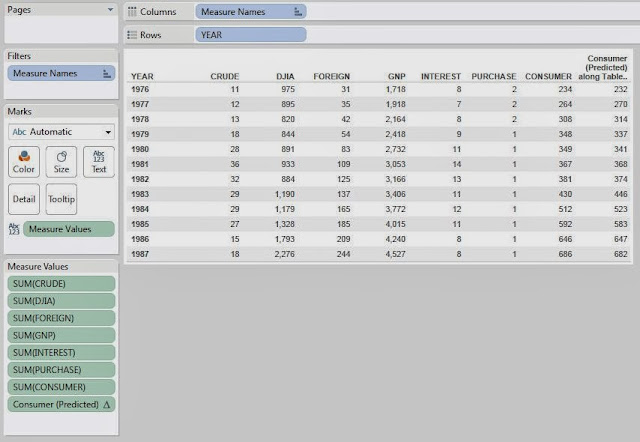Predicted Consumer Debt by Year (Text Table)
Just like in our previous post, this text table isn't very easy to read.  However, if you look closely, you will see that the predictions are significantly closer since we included all of the variables.  Now, we're stuck with another dilemma.  How do we see the results visually?  We can't have a seven-dimensional scatterplot.  Well, there are a couple of different ways.  First, let's look at what's called a Residual vs. Predicted plot.

Mathematically, the residuals are the differences between the actual value and the predicted value.  In the business world, you'll often hear this called a "Delta."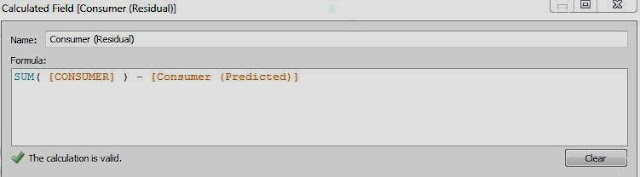Consumer (Residual)
Now, imagine that our model fit our data extremely well.  We wouldn't expect that the model fit perfectly.  However, we would expect the model to remove most of the "systematic" variation within the data, leaving only random noise.  This is precisely what this plot is designed to see.Residual vs. Predicted

Reading these types of charts is more art than science.  But, you can ask yourself one question, "Does the data form any significant pattern?"  We would say no.  Therefore, we believe that our model is a good fit for this data.  Now, let's visualize the data in its original context, by year.  Remember in the last post where we talked about "Prediction Intervals"?  We can make those here as well, using almost identical code.  The code for Consumer (Predicted Lower) is pasted below:

SCRIPT_REAL( "

## Defining Variables

cons <- .arg1
crud <- .arg2
djia <- .arg3
fore <- .arg4
gnp <- .arg5
inte <- .arg6
purc <- .arg7

## Fitting the Model

fit <- lm( cons ~ crud + djia + fore + gnp + inte + purc )

## Creating the Prediction Interval

dat <- data.frame(cbind(cons,crud,djia,fore,gnp,inte,purc))
predict(fit, dat, interval = 'prediction')[,2]
"
, SUM( [CONSUMER] ), SUM( [CRUDE] ), SUM( [DJIA] ), SUM( [FOREIGN] ),
SUM( [GNP] ), SUM( [INTEREST] ), SUM( [PURCHASE] ) )

To find the upper bound, you simply need to change the ,2 to a ,3.  Finally, let's plot our data.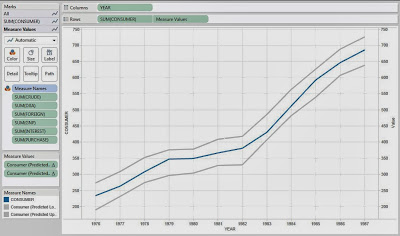Predicted Consumer Debt by Year (Line Chart)
As you can see, the bounds fit the actual data (blue line) very tightly and follow it as it increases over time.  Now, some of the more knowledgeable readers might say, "Multiple Regression requires uncorrelated observations, not time series data!"  You would be correct.  However, our goal here was simply to predict Consumer Debt.  We don't care about using the model to show correlation/causation between these variables.  We'll leave that to the econometricians.  Thanks for reading.  We hope you found this informative.